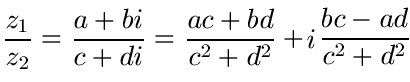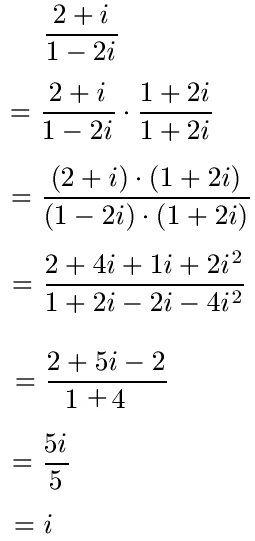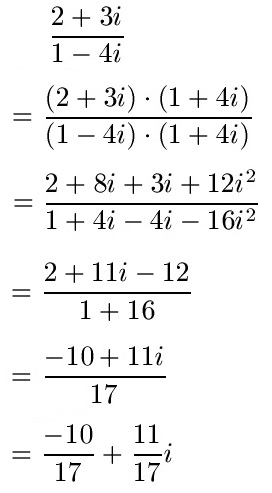# How can imaginary numbers be divided?

## Complex numbers division / divide

You will learn how to divide complex numbers in this article. I will briefly show the general context for the calculation, then some examples or tasks and give some general information. This article on complex number division is part of our math section.In the Complex Number Basics article, we covered some of the basics of complex numbers. This article is now about arithmetic with complex numbers, more precisely the division is dealt with. First the general context in brief, then examples.

General context:

There are numerous representations for the general representation of complex number division. So please don't be surprised if another source presents this differently. Afterwards we look at examples, which then show that the calculation can almost be carried out using methods known from school.Show:

### Complex numbers division examples

There is one more point that I need to address before giving examples. We need the so-called complex conjugate number to perform the division of complex fractions. What does that mean? Well, the complex conjugate number is mirror-symmetric to the real axis. You can get this very simply by reversing the sign in front of the imaginary part.

Examples conjugate complex number:

• The complex conjugate for 1 -2i is 1 + 2i.
• The complex conjugate for 3 + 4i is 3 - 4i.

To do the complex number division we will expand the fraction equal to complex conjugate. Hence these two examples.

example 1:

You want to calculate 2 + i divided by 1- 2i. First the invoice, then the explanations.As a first step we expand conjugate complex. As described above, we take the denominator and swap the sign. So 1 - 2i becomes 1 + 2i and we multiply this by the numerator and denominator. We multiply, as we already know from multiplying parentheses from school. If you still have problems here, please read the article Multiplying brackets. For the next step it is important to know that i2 = -1 is. This turns + 2i2 now -2 and from -4i2 becomes +4. We summarize further and abbreviate, the answer is 1i.

Example 2:

In the second example we want to calculate 2 + 3i divided by 1 - 4i.Here, too, expanding is initially conjugated complex. Since the denominator is 1 - 4i, this would be 1 + 4i. We multiply out and use the relationship i again2 = -1. Then we simplify and change the representation.

Complex Numbers Division Notes:

• For the conjugate complex number the sign of the imaginary part has to be reversed.
• One should always be aware that i2 = -1 must be used.
• Even with complex division, it is not allowed to divide by zero.
• The complex conjugate expansion makes the denominator real.